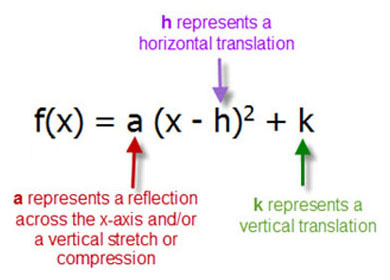4 years ago
laleonard254
Save
Edit
Host a game
Live GameLive
Homework
Solo Practice
Practice14 QuestionsShow answers
• Question 1
180 seconds
Q. Which transformation maps the graph of
f(x) = x2 to the graph of g(x) = (x + 4)2?
a reflection across the line x = -4
a reflection across the line y = -4
a translation shifting f(x) 4 units to the left
a translation shifting f(x) 4 units to the right
• Question 2
300 seconds
Q. Pablo throws a ball with a path of
h = -5(t - 2)2.
Maria throws a ball with a path of
h = -5(t - 2)2 + 10.
Which description is reasonable for the difference in the two equations?
Maria throws the ball 10 times harder than Pablo does.
Maria starts her ball at a height 10 feet higher than Pablo does.
Pablo throws the ball 10 times harder than Maria does.
Pablo starts his ball at a height 10 feet higher than Maria does.
• Question 3
60 secondsQ. Match the description to its equation.
Reflected over the x-axis
Stretched by a factor of 2
A
B
C
D
• Question 4
60 secondsQ. Match the description to its equation.
Moves up 2
Moves left 4
A
B
C
D
• Question 5
60 secondsQ. Match the description to its equation.
Moves right 7
A
B
C
D
• Question 6
60 secondsQ. Match the description to its equation.
- moves down 3
A
B
C
D
• Question 7
60 secondsQ. Match the equation to its description.
Reflected over x and up 4
Reflected over x and down 4
Reflected over x and right 4
Reflected over x and left 4
• Question 8
60 secondsQ. Match the equation to its description.
Stretched by 2 and up 4
Compressed by 2 and up 4
Stretched by 2 and left 4
Compressed by 2 and left 4
• Question 9
60 secondsQ. Match the equation to its description.
Stretched by 4 and up 4
Compressed by 4 and up 4
Stretched by 4 and left 4
Compressed by 4 and left 4
• Question 10
60 secondsQ. Match the description to its equation.
Moves down 1
Moves right 1
A
B
C
D
• Question 11
120 seconds
Q. Which list orders the quadratic functions from narrowest to widest?
y = x2
y = 4x2
y = 2x2 - 2
y = 0.5 x2
y = 0.5 x2
y = x2
y = 2x2 - 2
y = 4x2
y = 4x2
y = 2x- 2
y = x2
y = 0.5 x
y = 2x2 - 2
y = 4x2
y = 0.5x2
y = x2
• Question 12
120 seconds
Q. Which transformation will occur if f(x) = x2 is replaced with 2⋅f(x)?
Nothing happens, it stays the same.
Vertical stretch by a factor of 2.
Vertical translation up by 2 units.
Reflection across the x-axis.
• Question 13
120 seconds
Q. Which transformation will occur if f(x) = x2 is replaced with f(x) + 2?
Nothing happens, it stays the same.
Vertical stretch by a factor of 2.
Vertical translation up by 2 units.
Reflection across the x-axis.
• Question 14
120 seconds
Q. Which transformation will occur if f(x) = x2 is replaced with -f(x)?